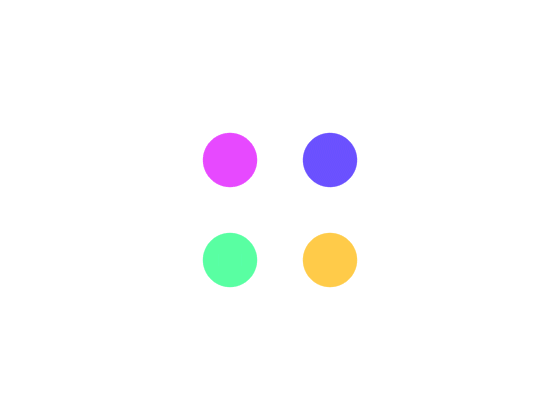#``````ob_start();//打开缓冲区
``````

``````ob_get_clean();//获取缓冲区并且清除
````````````\$content = "html内容";
\$output = ltrim(rtrim(preg_replace(array("/> *([^ ]*) *[ ]+\|反斜杠反斜杠|反斜杠1<'],\$content)));
``````

``````\$data = [];//存放pre标签内容
\$i = 0;//pre标签下标
htmlFilterPreCompress(\$data,\$content,\$i);

function htmlFilterPreCompress(\$data,\$content,\$index){
\$pre = "/<pre[反斜杠|反斜杠s反斜杠|反斜杠S]*?/";
if(preg_match(\$pre,\$content,\$m)){
//替换
\$content = str_replace(current(\$m),"[HTML_COMPRESS]{\$index}[/HTML_COMPRESS]",\$content);
\$data[\$index] = current(\$m);
\$index++;
//递归再次去检测一次
\$this->htmlFilterPreCompress(\$data,\$content,\$index);
}else{
\$output = ltrim(rtrim(preg_replace(array("/> *([^ ]*) *[ ]+反斜杠|反斜杠反斜杠|反斜杠1\$val){
\$output = str_replace("[HTML_COMPRESS]{\$key}[/HTML_COMPRESS]",\$val,\$output);
}
echo \$output;
}
}
``````

## 随便看看